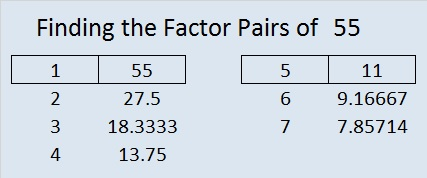# 55 and Square Roots for Third Grade

55 is a composite number. 55 = 1 x 55 or 5 x 11. Factors of 55: 1, 5, 11, 55. Prime factorization: 55 = 5 x 11.Sometimes 55 is a clue in the FIND THE FACTORS 1 – 12 puzzles. Even though it has other factors, the puzzle only uses 5 x 11 = 55.

——————————————————————————————-

What is the square root of a number? If you had a square made with 9 squares, how many squares would be on each side?Even a third grader can count 3 squares on each side. That is one way to explain why √9 = 3 to someone so young. Here’s another explanation:

1. 1 x 1 = 1, so √1 = 1
2. 2 x 2 = 4, so √4 = 2
3. 3 x 3 = 9, so √9 = 3
4. 4 x 4 = 16, so √16 = 4
5. 5 x 5 = 25, so √25 = 5
6. 6 x 6 = 36, so √36 = 6
7. 7 x 7 = 49, so √49 = 7
8. 8 x 8 = 64, so √64 = 8
9. 9 x 9 = 81, so √81 = 9
10. 10 x 10 = 100, so √100 = 10

These are the kinds of square roots that third grade students may need to know. When they get older they will learn about other square roots like √2 ≈ ±1.414, but third graders don’t need to worry about those yet.

On January 24 someone hurriedly typed “aquare roots for third grade” into a search engine, and a post from this blog came up. The person took ONE quick look and closed the post and the blog just as hurriedly. I’m not exactly sure what aquare or square roots for third grade means, but I felt bad that the blog disappointed this unknown person. I thought, “That person MAY have been searching for something that actually might be on the blog SOMEWHERE…..Perhaps a puzzle with perfect square clues would have been a welcome sight.” Hey, I can dream, can’t I?

To solve today’s puzzle (It actually has perfect square clues!), you will have to take the square roots of the clues, and place those square roots in the top row as well as the first column. After you write down all those square roots, figure out what numbers go in the empty cells so that the entire puzzle works as a multiplication table.These square roots may or may not be the square roots for third grade that person was looking for. Nevertheless, I have personally seen several bright THIRD grade students solve similar perfect square puzzles that I’ve made. This week’s puzzles are available in an excel file here. If you have a spreadsheet program on your computer, you can access it. If you enable editing in excel, you can type your answers directly onto the puzzle, and you can also easily print the puzzles. Here are the factors for last week’s level 1 puzzle:This site uses Akismet to reduce spam. Learn how your comment data is processed.